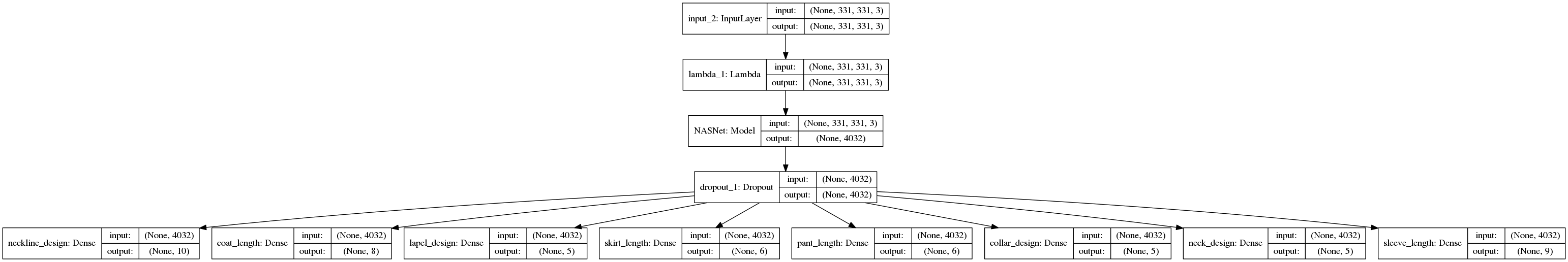# AI 多类分类(multi-class) and 多标签分类(mulit-label) and 多输出-多分类-多标签classification

sigmoidsoftmax是神经网络输出层使用的激活函数，分别用于两类判别和多类判别。

binary cross-entropycategorical cross-entropy是相对应的损失函数。

（详见Pattern Recognition and Machine Learning一书5.3章）

softmax有其特殊性，只用于最后一层。其它的激活函数都是单输入单输出的，softmax是多输入多输出的。

1. from keras.layers import Input,Dense
2. from keras.models import Sequential
3. model = Sequential()
4. model.add(Dense(10, activation="relu", input_shape=(10,))) <---现在激活层一般都用relu
5. model.add(Dense(5)) <---输出5类

## 多类别分类(Multiclass classification):

表示分类任务中有多个类别, 比如对一堆水果图片分类, 它们可能是橘子、苹果、梨等. 多类分类是假设每个样本都被设置了一个且仅有一个标签: 一个水果可以是苹果或者梨, 但是同时不可能是两者.
数据类别：[[1,0,0],[0,1,0],...] <---(3分类)

softmax层中的softmax 函数是logistic函数在多分类问题上的推广，它将一个N维的实数向量压缩成一个满足特定条件的N维实数向量。压缩后的向量满足两个条件：

• 向量中每个元素的的概率大小都在[0,1]
• 所有向量元素的概率和为1

import numpy as np

def Softmax_sim(x):
y = np.exp(x)
return y/np.sum(y)

x = np.array([1.0,2.0,3.0,4.0,1.0])
print(Softmax_sim(x))
#输出：[ 0.03106277 0.08443737 0.22952458 0.6239125 0.03106277]

from keras.layers import Input,Dense
from keras.models import Model

inputs = Input(shape=(10,))
hidden = Dense(units=10,activation='relu')(inputs)
output = Dense(units=5,activation='softmax')(hidden)

使用keres InceptionResNetV2分类，示例：

base_model = InceptionResNetV2(weights='imagenet', input_shape=(width, width, 3), include_top=False, pooling='max')

input_tensor = Input((width, width, 3))
x = input_tensor
x = Lambda(inception_resnet_v2.preprocess_input)(x)
x = base_model(x)
#x = GlobalAveragePooling2D()(x)
x = Dropout(0.6)(x)
x = Dense(5, activation='softmax', name='byz')(x)

model = Model(input_tensor, x)


## 多标签分类(Multilabel classification):

给每个样本一系列的目标标签. 可以想象成一个数据点的各属性不是相互排斥的(一个水果既是苹果又是梨就是相互排斥的), 比如一个文档相关的话题. 一个文本可能被同时认为是宗教、政治、金融或者教育相关话题.

数据类别：[[1,0,1],[0,1,0],[1,1,1],[0,0,0]...] <---(3标签分类，每个物品可以同时表示多类，预测得到可能是每个类的概率)

从<多类分类>中知道，通过使用softmax，我们可以清楚地选择标签4(或者选择更多标签)。但我们必须知道每个样本需要多少个标签，或者为概率选择一个阈值。这显然不是我们想要的，因为样本属于每个标签的概率应该是独立的（也就是自己和自己比，而不是和所有的属性相比）。

使用keres InceptionResNetV2分类，示例：


base_model = InceptionResNetV2(weights='imagenet', input_shape=(width, width, 3), include_top=False, pooling='max')

input_tensor = Input((width, width, 3))
x = input_tensor
x = Lambda(inception_resnet_v2.preprocess_input)(x)
x = base_model(x)
#x = GlobalAveragePooling2D()(x)
x = Dropout(0.6)(x)
x = Dense(label_count, activation='sigmoid', name='byz')(x) #<-----------区别在这里

model = Model(input_tensor, x)

# 所的结果可能如：

# ['blending', 'knit', 'silk', 'leather', 'denim', 'cotton', 'chemical fiber', 'flax', 'woolen']
# [0.8194268, 0.318167, 0.37516874, 0.17264916, 0.08276224, 0.80080295, 0.55450606, 0.1958817, 0.3615162]

# 可以看到得到的结果是各自的类别概率，而不是加起来为1



## 多输出回归(Multioutput classification):

给每个样本一系列的目标值. 可以被想象成对每个数据点预测多个属性, 比如在某个定位的风向和风速

## 多输出-多分类-多标签 分类(Multioutput-multiclass classification) 和 多任务分类(Multi-task classification):

意味着一个单一的评估器需要处理多个联合分类任务. 这是多标签分类任务(只考虑二院分类)和多类分类任务的推广, 输出格式是2d阵列.

• 每一个输出变量的标签可以是不同的. 比如一个样本的第一输出变量可以是有限类别中是pear的概率值, 第二个输出变量可能是有限颜色中是blue或者green的概率.
• 这意味着任意的支持多输出多类或者多任务分类任务的分类器, 均支持作为一种特殊情况的多标签分类任务. 多任务分类与多输出分类任务相似, 但是有不同的模型公式.

ps: sigmoid函数之所以在之前很长一段时间作为神经网络激活函数（现在大家基本都用Relu了），一个很重要的原因是sigmoid函数的导数很容易计算，可以用自身表示，所有我们使用它得到我们想要的。

使用keres InceptionResNetV2分类，示例：


base_model = InceptionResNetV2(weights='imagenet', input_shape=(width, width, 3), include_top=False, pooling='max')

input_tensor = Input((width, width, 3))
x = input_tensor
x = Lambda(inception_resnet_v2.preprocess_input)(x)
x = base_model(x)
#x = GlobalAveragePooling2D()(x)
x = Dropout(0.6)(x)
#x = [Dense(label_count[name], activation='sigmoid', name=name)(x) for name in label_counts] #softmax
# 使用不同的类别概率输出方式：
xx= []
for name in label_counts:
if name == 'XXX' or name == 'YYY':
activation = 'sigmoid'
xx.append(Dense(label_count[name], activation=activation, name=name)(x))
else:
activation = 'softmax'
xx.append(Dense(label_count[name], activation=activation, name=name)(x))

model = Model(input_tensor, xx)

# 所得结果可能如：

# [一系列类别...] --(概率独立
# [0.8194268, 0.318167, 0.37516874,0.80080295, 0.55450606, 0.1958817, 0.3615162]
#
# [一系列类别...] --(概率不独立
[0.012616142, 0.898532, 0.020602752, 0.011825822, 0.05642339]
#
# [一系列类别...] --(概率独立
# [0.3250507, 0.03250401, 0.019756323,0.19708893, 0.28087845, 0.06590339, 0.24679133, 0.08187613, 0.36806807, 0.07590864, 0.1500308, 0.02745669]



from keras.models import Model
from keras.layers import Input,Dense

inputs = Input(shape=(10,))
hidden = Dense(units=10,activation='relu')(inputs)
output = Dense(units=5,activation='sigmoid')(hidden)
model.compile(optimizer='adam', loss='binary_crossentropy', metrics=['accuracy'])07-21640
04-223225
05-053687
08-041399
08-105万+
06-08767
11-284259
08-302万+
08-10340
04-232563
03-191万+
07-041万+
12-249325
05-104万+
08-093万+
04-081万+
03-067600
11-082639